# Thread: need help differentiating cos^(4)3t sin3 tdt

1. ## need help differentiating cos^(4)3t sin3 tdt

i need help differentiating this fcn
i think u is 3t?
but im lost as to where to go from there. could someone walk me through it?
thanks!

2. Wait, differentiate or integrate?
I'll assume integration.

cos^(4)3t sin3t dt

You got the first step right. take u = 3t -> du = 3dt -> du/3 = dt. You can pull the 1/3 constant out of the integral after substitution

(1/3) * the integral cos^(4)u sinu du.

Now, use substitution again for this new integral. In this case, the substitution is simple, but in other trig forms like the one above, you may have to use some identities before substitution.

let v = cos u
dv = -sin u du
-dv = sin u du

You can pull the negative sign out of the integral after substitution as you had done with 1/3

-(1/3) * the integral of v^4 dv

Now integrate

-(1/3) * v^5/5
-(1/3) * cos^(5) u /5 (substitute back into v)
-(1/3) * cos^(5) 3t /5 (substitute back into u)

Hope that helps.

3. If you DID mean differentiating like you said...

(And if it says $\displaystyle \cos^4(3t)\ \sin(3t)$)

...then maybe try completing this...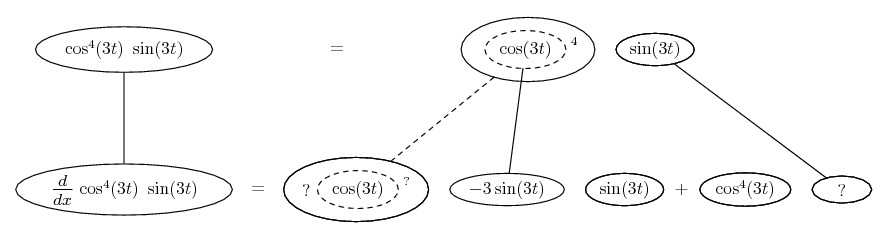Straight continuous lines differentiate downwards with respect to x, and the straight dashed line with respect to the dashed balloon expression - for the sake of the chain rule, the general pattern being...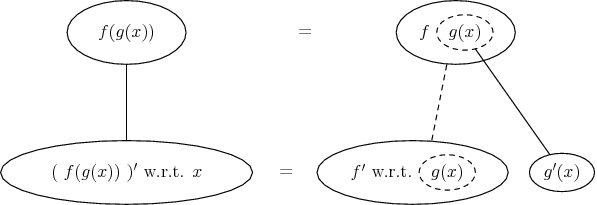Here the chain rule is wrapped inside the product rule, generally...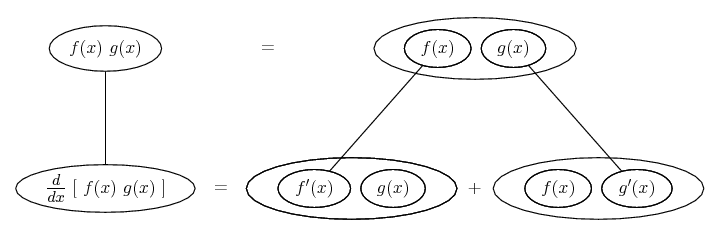... more visible here if we enclose some of the balloons in some more...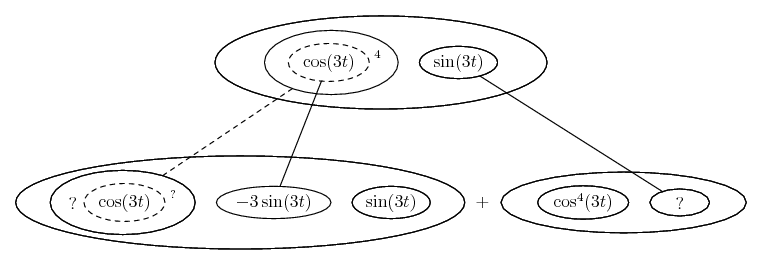Don't integrate - balloontegrate!
Balloon Calculus: worked examples from past papers

4. And if you are integrating, a picture can also be useful (see what you think)...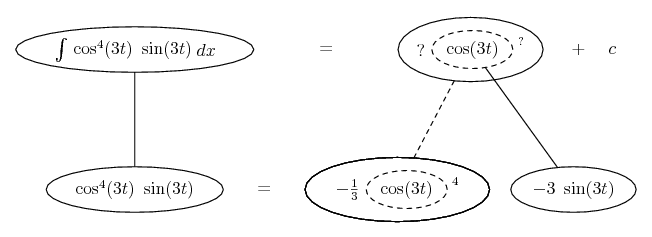Don't integrate - balloontegrate!
Balloon Calculus: worked examples from past papers

,

,

,

,

,

,

,

,

,

# cos^4 3t

Click on a term to search for related topics.

#### Search Tags

cos43t, differentiating, sin3, tdt